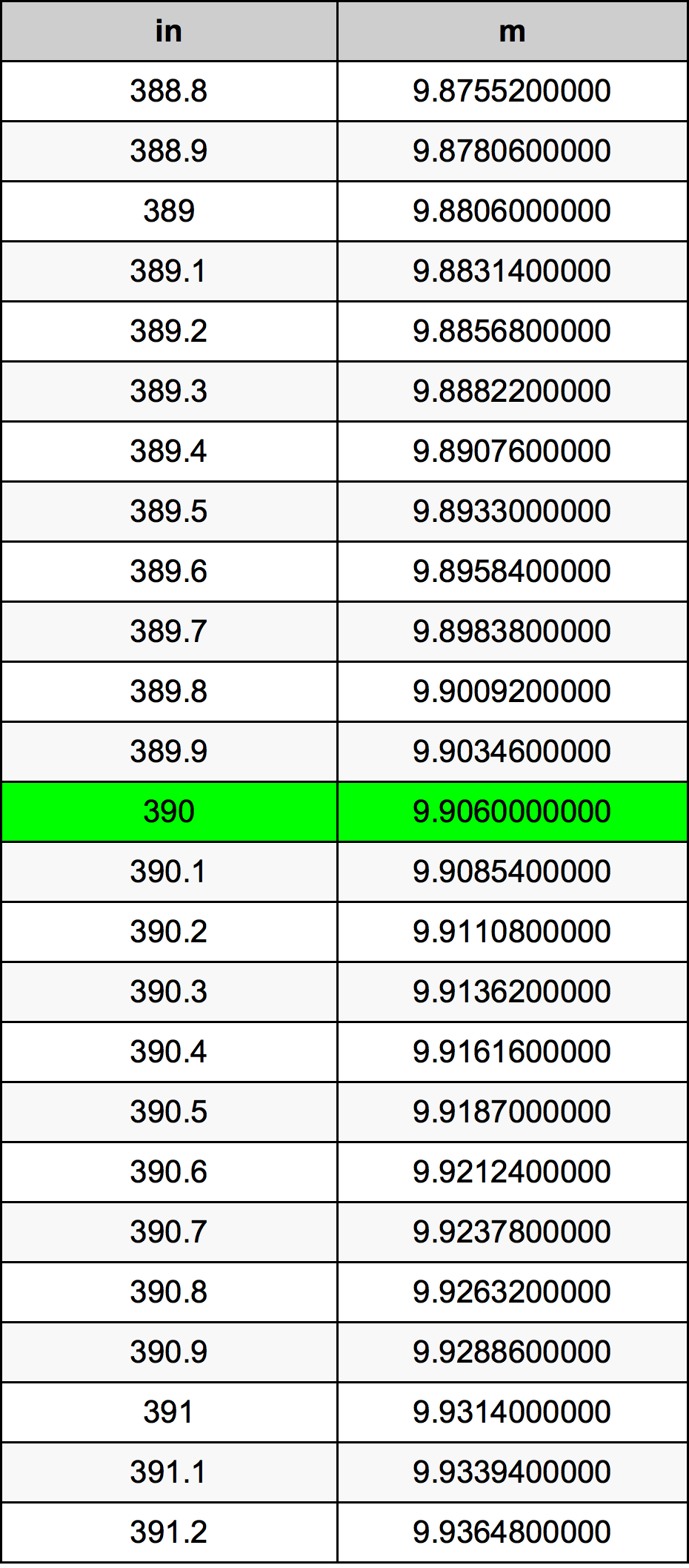Inches To Meters

# 390 in to m390 Inches to Meters

in
=
m

## How to convert 390 inches to meters?

 390 in * 0.0254 m = 9.906 m 1 in
A common question is How many inch in 390 meter? And the answer is 15354.3307087 in in 390 m. Likewise the question how many meter in 390 inch has the answer of 9.906 m in 390 in.

## How much are 390 inches in meters?

390 inches equal 9.906 meters (390in = 9.906m). Converting 390 in to m is easy. Simply use our calculator above, or apply the formula to change the length 390 in to m.

## Convert 390 in to common lengths

UnitLength
Nanometer9906000000.0 nm
Micrometer9906000.0 µm
Millimeter9906.0 mm
Centimeter990.6 cm
Inch390.0 in
Foot32.5 ft
Yard10.8333333333 yd
Meter9.906 m
Kilometer0.009906 km
Mile0.006155303 mi
Nautical mile0.0053488121 nmi

## What is 390 inches in m?

To convert 390 in to m multiply the length in inches by 0.0254. The 390 in in m formula is [m] = 390 * 0.0254. Thus, for 390 inches in meter we get 9.906 m.

## 390 Inch Conversion Table## Alternative spelling

390 Inches to Meter, 390 Inches in Meter, 390 Inches to m, 390 Inches in m, 390 in to m, 390 in in m, 390 Inch to m, 390 Inch in m, 390 Inch to Meters, 390 Inch in Meters, 390 in to Meter, 390 in in Meter, 390 Inches to Meters, 390 Inches in Meters